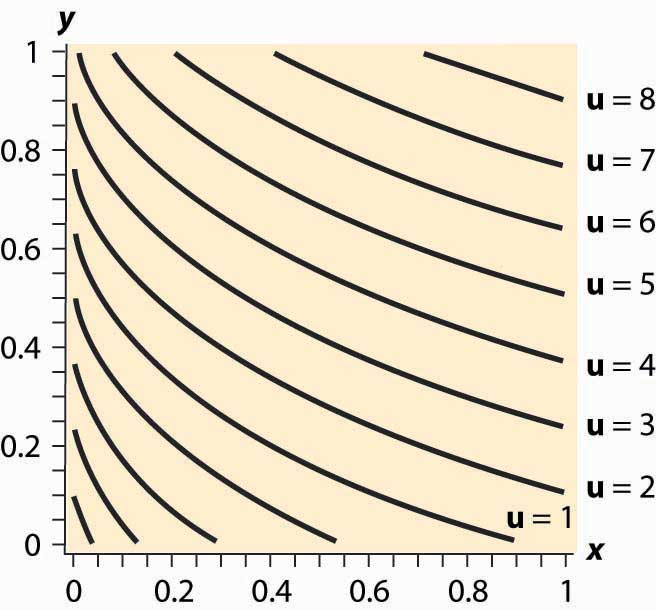# 12.7: Mathematical Cleanup

•• Anonymous
• LibreTexts
$$\newcommand{\vecs}{\overset { \rightharpoonup} {\mathbf{#1}} }$$ $$\newcommand{\vecd}{\overset{-\!-\!\rightharpoonup}{\vphantom{a}\smash {#1}}}$$$$\newcommand{\id}{\mathrm{id}}$$ $$\newcommand{\Span}{\mathrm{span}}$$ $$\newcommand{\kernel}{\mathrm{null}\,}$$ $$\newcommand{\range}{\mathrm{range}\,}$$ $$\newcommand{\RealPart}{\mathrm{Re}}$$ $$\newcommand{\ImaginaryPart}{\mathrm{Im}}$$ $$\newcommand{\Argument}{\mathrm{Arg}}$$ $$\newcommand{\norm}{\| #1 \|}$$ $$\newcommand{\inner}{\langle #1, #2 \rangle}$$ $$\newcommand{\Span}{\mathrm{span}}$$ $$\newcommand{\id}{\mathrm{id}}$$ $$\newcommand{\Span}{\mathrm{span}}$$ $$\newcommand{\kernel}{\mathrm{null}\,}$$ $$\newcommand{\range}{\mathrm{range}\,}$$ $$\newcommand{\RealPart}{\mathrm{Re}}$$ $$\newcommand{\ImaginaryPart}{\mathrm{Im}}$$ $$\newcommand{\Argument}{\mathrm{Arg}}$$ $$\newcommand{\norm}{\| #1 \|}$$ $$\newcommand{\inner}{\langle #1, #2 \rangle}$$ $$\newcommand{\Span}{\mathrm{span}}$$

## LEARNING OBJECTIVES

1. Are there important details that haven’t been addressed in the presentation of utility maximization?
2. What happens when consumers buy none of a good?

Let us revisit the maximization problem considered in this chapter to provide conditions under which local maximization is global. The consumer can spend M on either or both of two goods. This yields a payoff of $$\begin{equation}h(x)=u(x, M-p X x p Y)\end{equation}$$. When is this problem well behaved? First, if h is a concave function of x, which implies h ″ (x)≤0, The definition of concavity is such that h is concave if 0 < a < 1 and for all x, y, $$\begin{equation}h(a x+(1-a) y) \geq a h(x)+(1-a) h(y)\end{equation}$$. It is reasonably straightforward to show that this implies the second derivative of h is negative; and if h is twice differentiable, the converse is true as well. then any solution to the first-order condition is, in fact, a maximum. To see this, note that h ″ (x)≤0 entails h ′ (x) is decreasing. Moreover, if the point x* satisfies h ′ (x*)=0, then for $$\begin{equation}x \leq x^{*}, h^{\prime}(x) \geq 0 ; \text { and for } x \geq x^{*}, h^{\prime}(x) \leq 0\end{equation}$$, because h ′ (x) gets smaller as x gets larger, and h ′ (x*)=0. Now consider x ≤ x*. Since h ′ (x)≥0, h is increasing as x gets larger. Similarly, for x ≥ x*, h ′ (x)≤0, which means that h gets smaller as x gets larger. Thus, h is concave and h ′ (x*)=0 means that h is maximized at x*.

Thus, a sufficient condition for the first-order condition to characterize the maximum of utility is that h ″ (x)≤0 for all x, pX, pY, and M. Letting z= p X p Y , this is equivalent to u 11 −2z u 12 + z 2 u 22 ≤0 for all z > 0.

In turn, we can see that this requires (i) u11 ≤ 0 (z = 0), (ii) u22 ≤ 0 (z→∞), and (iii) u 11 u 22 − u 12 ≥0 ( z= u 11 u 22 ). In addition, since

\begin{equation}-(u 11+2 z u 12+z 2 u 22)=(-u 11-z-u 22) 2+2 z(u 11 u 22-u 12)\end{equation}

(i), (ii), and (iii) are sufficient for u11 +2zu 12 + z2u 22 ≤0.

Therefore, if (i) u11 ≤ 0, (ii) u22 ≤ 0, and (iii) u 11 u 22 − u 12 ≥0, a solution to the first-order conditions characterizes utility maximization for the consumer.

When will a consumer specialize and consume zero of a good? A necessary condition for the choice of x to be zero is that the consumer doesn’t benefit from consuming a very small x ; that is, h ′ (0)≤0. This means that

\begin{equation}h^{\prime}(0)=u 1(0, M p Y)-u 2(0, M p Y) p X p Y \leq 0\end{equation}

or

\begin{equation}\text { u } 1(0, \mathrm{MpY}) \text { u } 2(0, \mathrm{MpY}) \leq \mathrm{pX} \mathrm{pY}\end{equation}

Moreover, if the concavity of h is met, as assumed above, then this condition is sufficient to guarantee that the solution is zero. To see this, note that concavity of h implies h ′ is decreasing. Combined with h ′ (0)≤0, this entails that h is maximized at 0. An important class of examples of this behavior is quasilinear utility. Quasilinear utility comes in the form u(x, y) = y + v(x), where v is a concave function ( v ″ (x)≤0 for all x). That is, quasilinear utility is utility that is additively separable.

Figure 12.14 Quasilinear isoquantsThe procedure for dealing with corners is generally this. First, check concavity of the h function. If h is concave, we have a procedure to solve the problem; when h is not concave, an alternative strategy must be devised. There are known strategies for some cases that are beyond the scope of this text. Given h concave, the next step is to check the endpoints and verify that h ′ (0)>0 (for otherwise x = 0 maximizes the consumer’s utility) and h ′ ( M p X )<0 (for otherwise y = 0 maximizes the consumer’s utility). Finally, at this point we seek the interior solution h ′ (x)=0. With this procedure, we can ensure that we find the actual maximum for the consumer rather than a solution to the first-order conditions that don’t maximize the consumer’s utility.

## Key Takeaways

• Conditions are available that ensure that the first-order conditions produce a utility maximum.
• With convex preferences, zero consumption of one good arises when utility is decreasing in the consumption of one good, spending the rest of income on the other good.

## EXERCISE

1. Demonstrate that the quasilinear consumer will consume zero X if and only if $$\begin{equation}v^{\prime}(0) \leq p x p y\end{equation}$$, and that the consumer instead consumes zero Y if $$\begin{equation}v^{\prime}(M p X) \geq p x p y\end{equation}$$. The quasilinear utility isoquants, for $$\begin{equation}v(x)=(x+0.03) 0.3\end{equation}$$, are illustrated in Figure 12.14 "Quasilinear isoquants". Note that, even though the isoquants curve, they are nonetheless parallel to each other.

This page titled 12.7: Mathematical Cleanup is shared under a CC BY-NC-SA license and was authored, remixed, and/or curated by Anonymous.